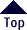By definition: the theory of Reality, its principles,  and its corollaries, must be simple. The most simple is the most complex;  thus . . . the most complex is  the most simple.
 The BRUNARDOT THEOREM (A Proof of One and the Unimetric Structure of Reality)
 v = ep2 for the ellipse: c2 = 2v2 - s2
 The Brunardot Theorem states that when: v = ep2 for the ellipse c2 = 2v2 - s2 a certain class of ellipses is created that determines the fundamental principles of number theory and Nature; where, c = a major chord; e = epsilon, the Elliptical Constant; p = a perigee; s = a soliton; and v = a vector. A corollary states that: p, s, v, and a, the apogee,  are the first four terms of a generalized Fibonacci series. When p and s are Natural integers the Brunardot Golden Corollaries result when the terms of said generalized Fibonacci series are those of the Brunardot Series. The Brunardot Series is a generalized Fibonacci series that has a Natural Integer for the second term and the third term is the square of the first.
 The formula: c2 = 2v2 - s2 describes a Fibonacci ellipse, where "c" is the length referred to as the Brunardot chord. "v" is the length referred to as the vector; and,
 "s" is the length referred to as the soliton; A Conceptual ellipse (CE) is a Fibonacci ellipse, which is generated for every Natural integer value of "Tau"; and, all Conceptual ellipses have a unique Natural Prime number for the diagonal, "d"; and, the following segments, as is the diagonal, are all Natural integers with values expressed in Natural Units, "U":
 Light, "l"; the perigee, "p"; the radius, "r";
 the soliton, "s"; the vector, "v"; and, the square of the Brunardot chord, "c."
 Most notably!  . . . four basic components of every Conceptual ellipse, (CE), are consecutive terms of a Fibonacci series.  The components, in sequence, are: the perigee, "p"; the soliton, "s"; the vector, "v"; and, the apogee, "a." A Brunardot ellipse (BE) is defined as:
 Any Conceptual ellipse (CE) that has the force, "F," as a Natural integer.
 Brunardot Harmonic Ellipses (BHE) are defined as:
 An unending series of Brunardot ellipses (BE) that are generated by any, single, Natural integer; and, have the vector "v" and the radius "r" as squares of integers; and with the diagonal "d," Brunardot Integer "i," and perigee "p" as Natural Prime numbers.
 Conceptual triangles describe Conceptual ellipses, which describe Conceptual ellipsoids that are generated by a combination of simple and complex sinusoidal oscillations with a diagonal that is a Natural Prime number.  Again, when the force, "F," is a Natural integer, the Conceptual ellipsoid becomes a Pulsoid, which underlies the unimetry (geometry) of the first essence of mass.

Click below to See the:
Conceptual ellipse and Brunardot ellipse Tables:

 E-mail :  Brunardot@HiPriority.com
 There is one Universe.
 It is perpetual, in equilibrium; and,
 a manifestation of the . . . Unified Concept;
 also,
 are a single discipline, which proclaims the
 perpetuity and nexus of Life; such is
 . . . Conceptualism.Terms of:  ©Copyright 1999-2007 by Brunardot
 Website Design by:   CONCEPTUAL  Applications a division of WebComm21

000105 0:01 am